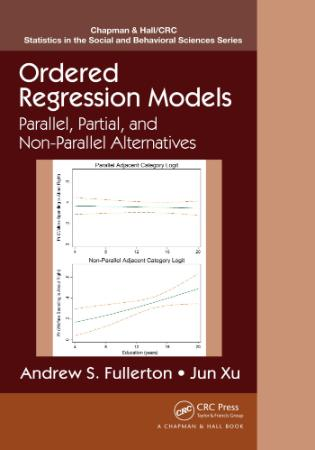# Ordered Regression Models Parallel, Partial, and Non Parallel Alternatives

#### Category: Uncategorized

DescriptionOrdered Regression Models Parallel, Partial, and Non Parallel Alternatives
pdf | 4.58 MB | English | Author :Fullerton, Andrew S.,Xu, Jun | B01DVTEUUW | 2016 | Chapman and Hall/CRC; 1 edition

Book Description :

Estimate and Interpret Results from Ordered Regression Models

Ordered Regression Models: Parallel, Partial, and Non-Parallel Alternatives presents regression models for ordinal outcomes, which are variables that have ordered categories but unknown spacing between the categories. The book provides comprehensive coverage of the three major classes of ordered regression models (cumulative, stage, and adjacent) as well as variations based on the application of the parallel regression assumption.

The authors first introduce the three "parallel" ordered regression models before covering unconstrained partial, constrained partial, and nonparallel models. They then review existing tests for the parallel regression assumption, propose new variations of several tests, and discuss important practical concerns related to tests of the parallel regression assumption. The book also describes extensions of ordered regression models, including heterogeneous choice models, multilevel ordered models, and the Bayesian approach to ordered regression models. Some chapters include brief examples using Stata and R.

This book offers a conceptual framework for understanding ordered regression models based on the probability of interest and the application of the parallel regression assumption. It demonstrates the usefulness of numerous modeling alternatives, showing you how to select the most appropriate model given the type of ordinal outcome and restrictiveness of the parallel assumption for each variable.

Web Resource
More detailed examples are available on a supplementary website. The site also contains JAGS, R, and Stata codes to estimate the models along with syntax to reproduce the results.

Category : | Probability & Statistics, Probability & Statistics

Hosters
Rapidgator | Nitroflare | DropApk

https://rapidgator.net/file/9169654e55fa18c6667963e9a0bf404c/Ordered.Regression.Models.Parallel.Partial.and.Non.Parallel.Alternatives.pdf
http://nitroflare.com/view/CB3E542FE4531B5/Ordered.Regression.Models.Parallel.Partial.and.Non.Parallel.Alternatives.pdf
https://dropapk.to/f0dghiij35qv/Ordered.Regression.Models.Parallel.Partial.and.Non.Parallel.Alternatives.pdf

7793 dl's @ 3524 KB/s
9295 dl's @ 2980 KB/s
8753 dl's @ 3922 KB/s

Search More...
Ordered Regression Models Parallel, Partial, and Non Parallel AlternativesRelated Books